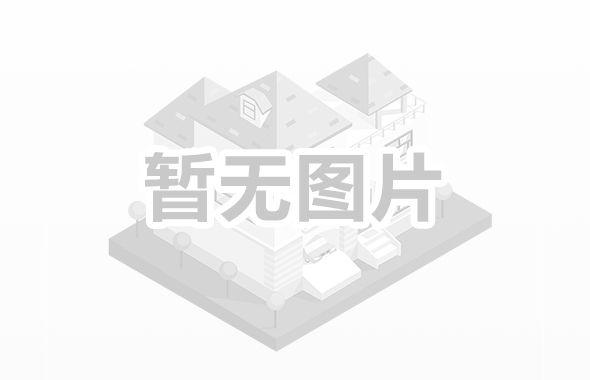# 117. 填充每个节点的下一个右侧节点指针 II

``````class Solution {

public Node connect(Node root) {
if (root != null) {
if (root.left != null) {
//左子节点的next
root.left.next = this.leftChildNode(root, root.left);
}
if (root.right != null) {
//右子节点的next
if (root.next != null) {
root.right.next = this.leftChildNode(root.next, root.right);
}
}
if (root.right != null) {
//递归右子节点
this.connect(root.right);
}
if (root.left != null) {
//递归左子节点
this.connect(root.left);
}
}
return root;
}

/**
* 获取node节点的子辈中，仅次于left节点的节点
*
* @param node
* @return
*/
private Node leftChildNode(Node node, Node left) {
if (node != null) {
//按照优先级，左，右，next的左右顺序返回，直到都为null
if (node.left != null && node.left != left) {
//因为node节点一定是left节点的父节点或者父节点的兄弟
//所以是否可以返回node节点的left节点判断依据是是不是等于left
return node.left;
} else if (node.right != null) {
return node.right;
} else {
return this.leftChildNode(node.next, left);
}
}
return null;
}

}
``````